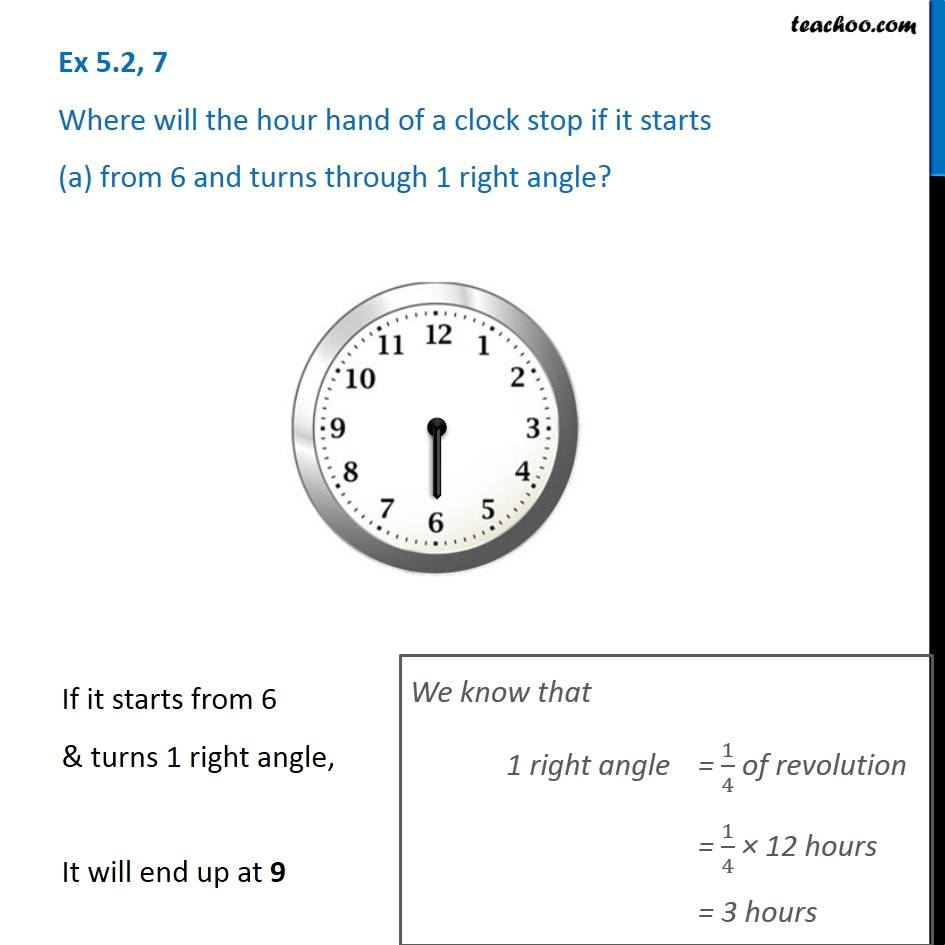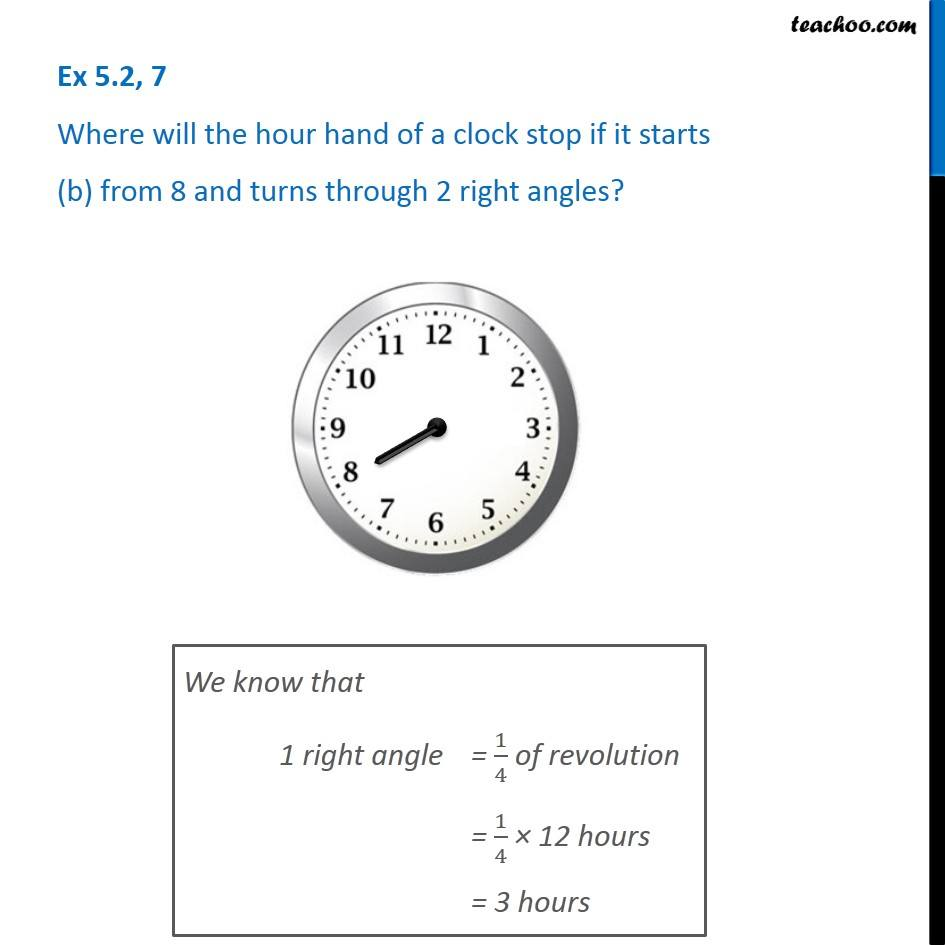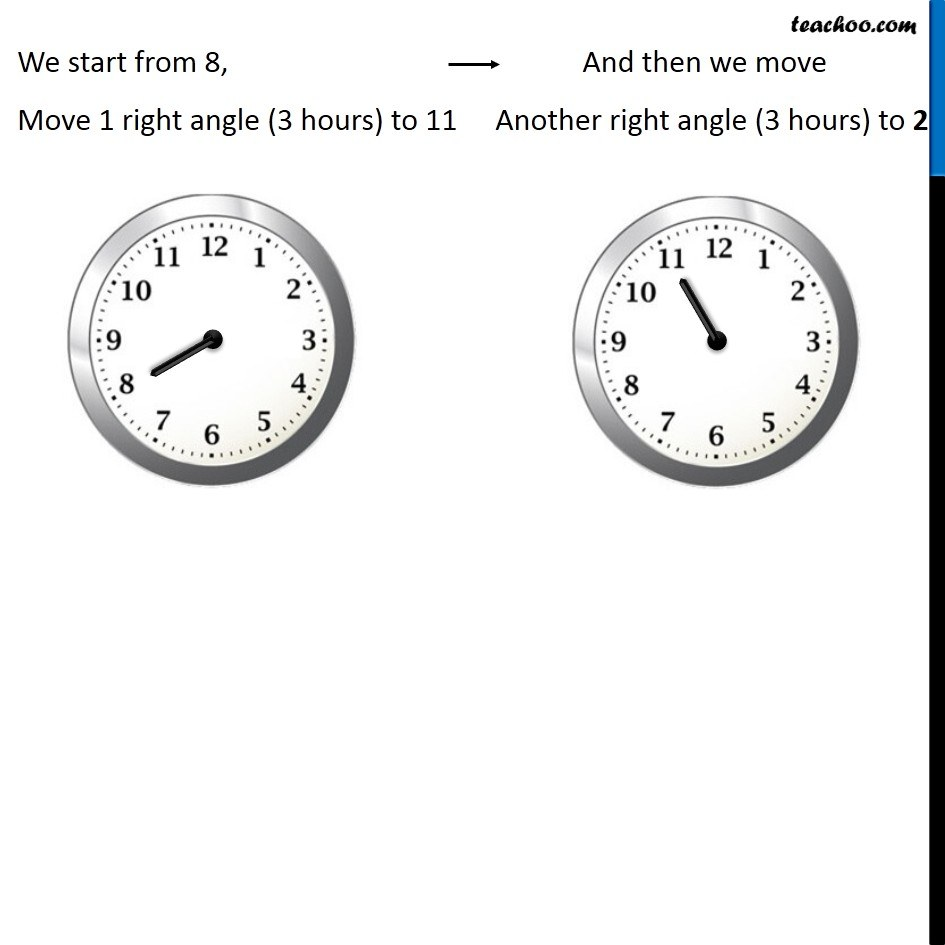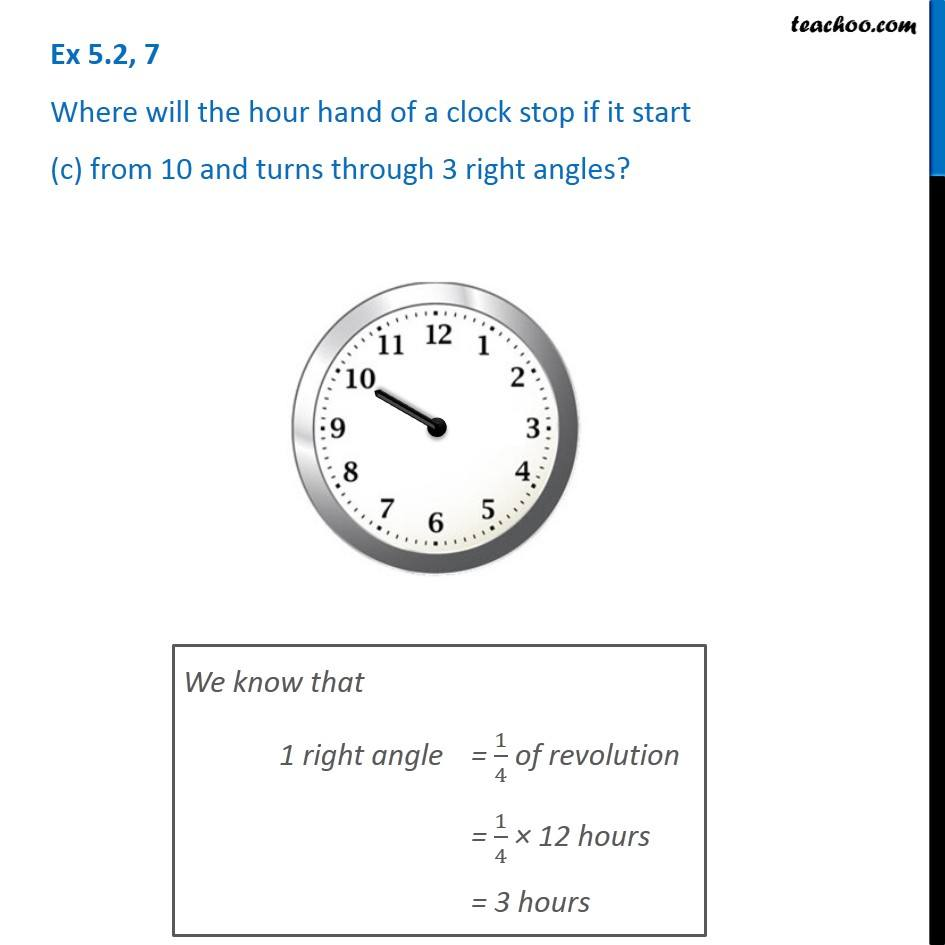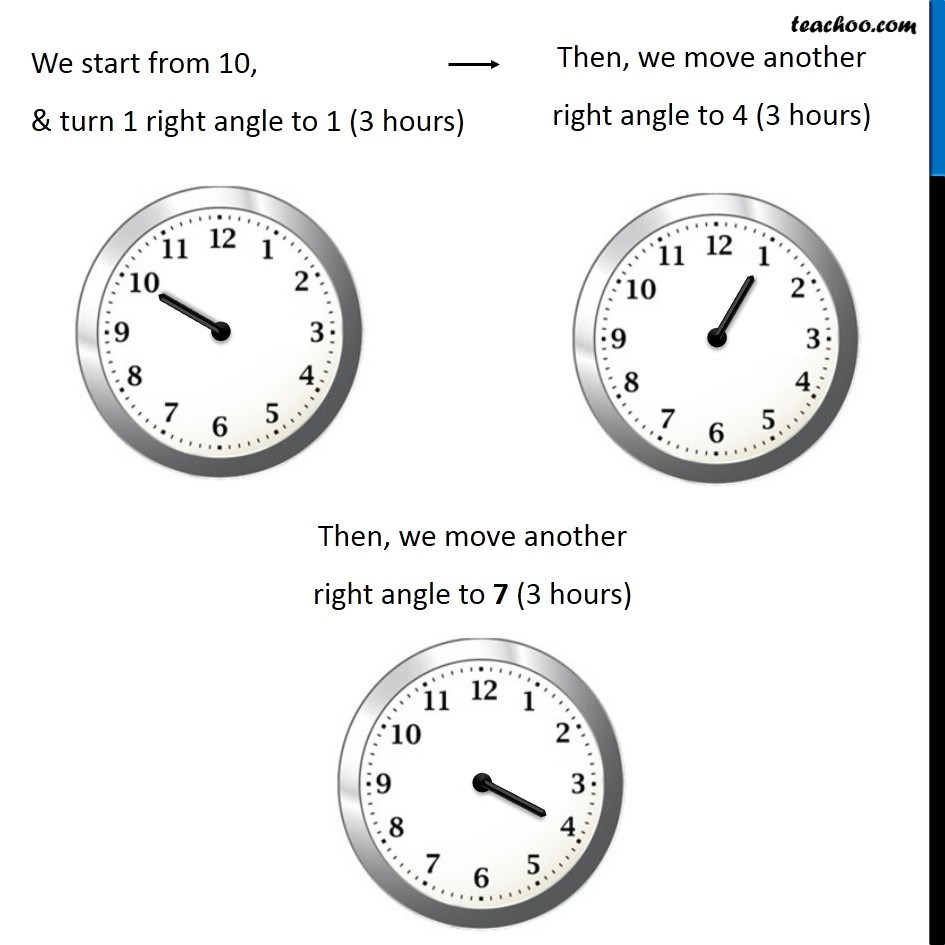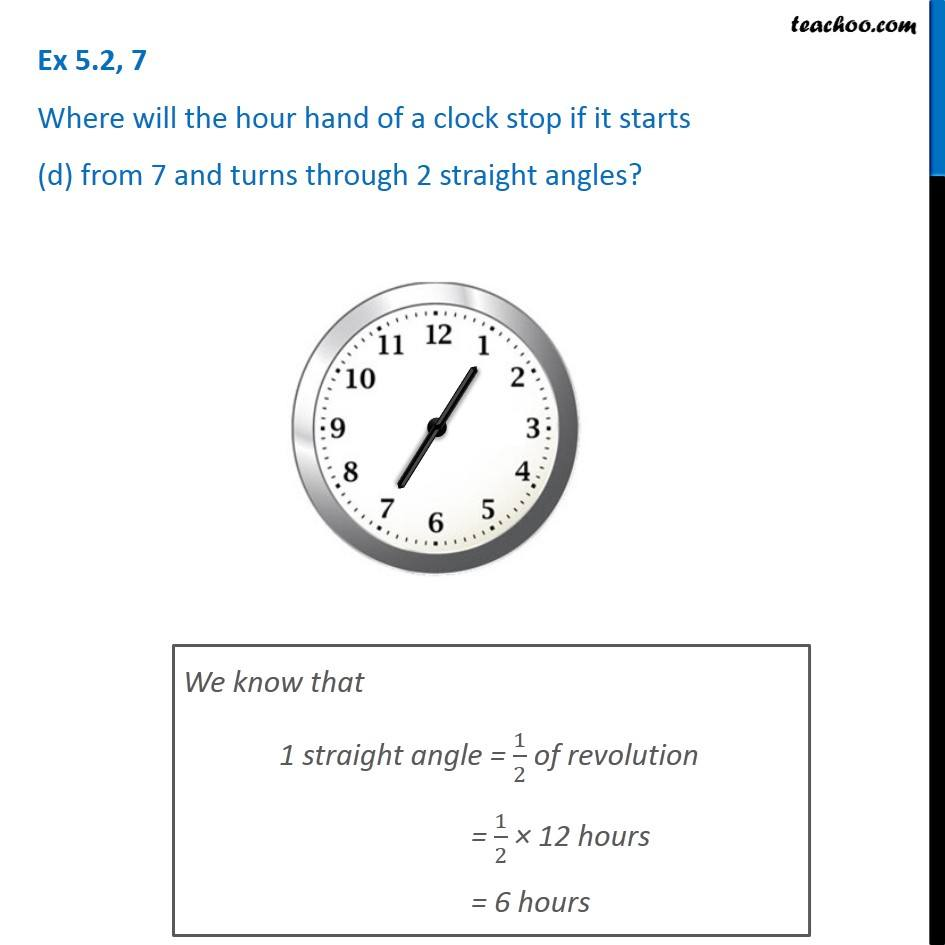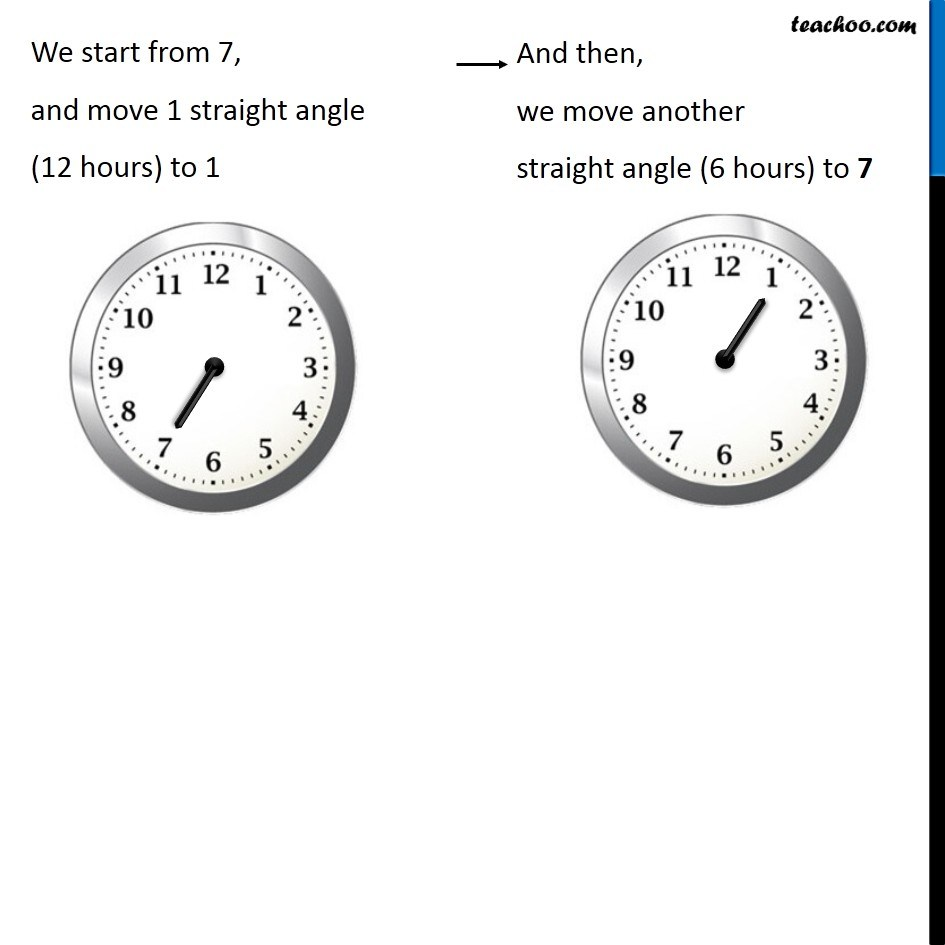Subscribe to our Youtube Channel - https://you.tube/teachoo

1. Chapter 5 Class 6 Understanding Elementary Shapes
2. Serial order wise
3. Ex 5.2

Transcript

Ex 5.2, 7 Where will the hour hand of a clock stop if it starts (a) from 6 and turns through 1 right angle? If it starts from 6 & turns 1 right angle, It will end up at 9 We know that 1 right angle = 1/4 of revolution = 1/4 × 12 hours = 3 hours Ex 5.2, 7 Where will the hour hand of a clock stop if it starts (b) from 8 and turns through 2 right angles? We know that 1 right angle = 1/4 of revolution = 1/4 × 12 hours = 3 hours We start from 8, Move 1 right angle (3 hours) to 11 And then we move Another right angle (3 hours) to 2 Ex 5.2, 7 Where will the hour hand of a clock stop if it start (c) from 10 and turns through 3 right angles? We know that 1 right angle = 1/4 of revolution = 1/4 × 12 hours = 3 hours We start from 10, & turn 1 right angle to 1 (3 hours) Then, we move another right angle to 4 (3 hours) Then, we move another right angle to 7 (3 hours) Ex 5.2, 7 Where will the hour hand of a clock stop if it starts (d) from 7 and turns through 2 straight angles? We know that 1 straight angle = 1/2 of revolution = 1/2 × 12 hours = 6 hours We start from 7, and move 1 straight angle (12 hours) to 1 We start from 7, and move 1 straight angle (12 hours) to 1

Ex 5.2